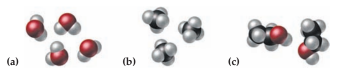Introductory Chemistry - 5 Edition - Chapter 5 - Problem 91p
Register Now

Join StudySoup

Get Full Access to Introductory Chemistry - 5 Edition - Chapter 5 - Problem 91p9780321910295

# Solved: Specify the number of hydrogen atoms (white) represented in each set of

Introductory Chemistry | 5th Edition

Problem 91P

Specify the number of hydrogen atoms (white) represented in each set of molecular models:Accepted Solution
Step-by-Step Solution:
Step 1 of 3

Problem 91P

Specify the number of hydrogen atoms (white) represented in each set of molecular models:

(a)(b)(c)Solution 91P:

Here, we are going to calculate the number of hydrogen  atoms present in each  set of molecular models.

Atom is the smallest tiny representation of an atom.

Chemical formula is the representation of the number of atoms present in the molecule or molecular compound .

(a)It is a model of Water molecule (H2O)

Total number of Hydrogen atoms in (a) = 2 x 4 =8

(b)It is a model of methane molecule (CH4)

Total number of Hydrogen atoms in (b) = 3 x 4 =12

(c)Total number of Hydrogen atoms in (c) = 2 x 6 =12

###### Chapter 5, Problem 91P is Solved

Step 2 of 3

Step 3 of 3

Unlock Textbook Solution